# Problems of the Week

Contribute a problem

# 2018-03-26 Basic

The red and blue areas in the following $8 \times 8$ square are equal: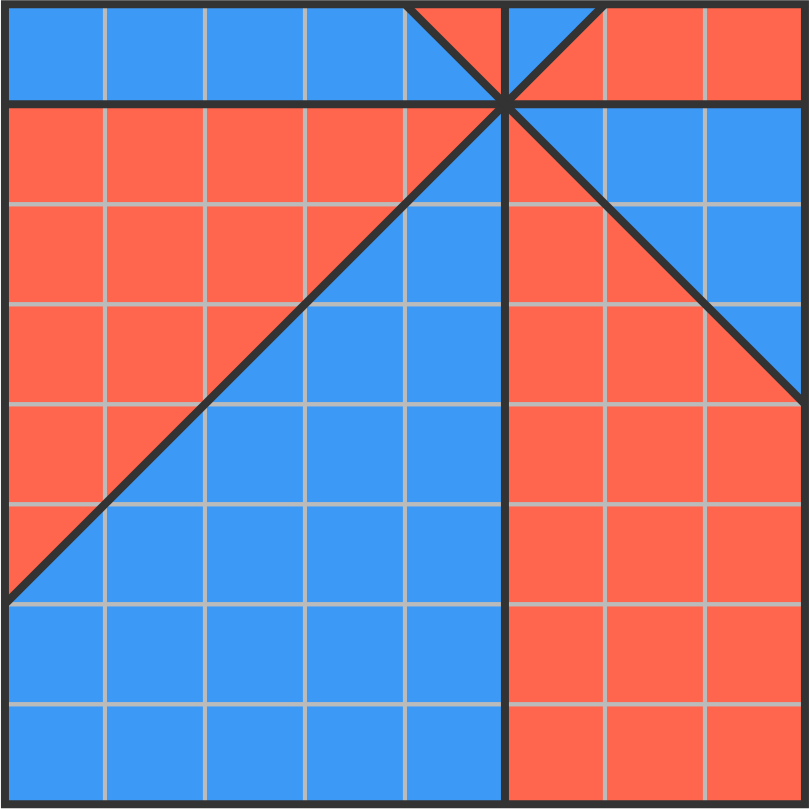Are the red and blue areas still equal in the circle below?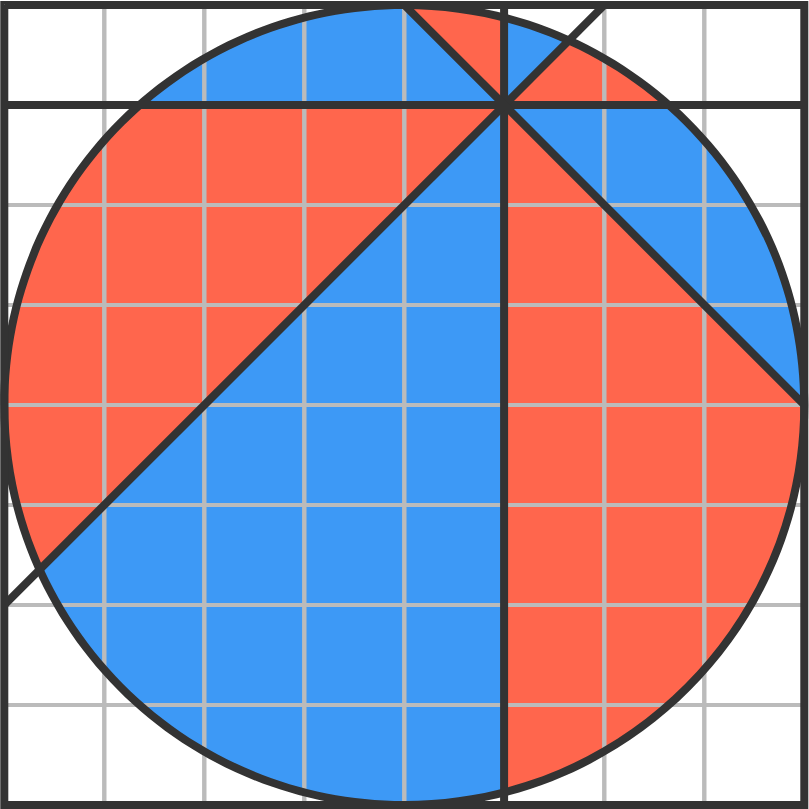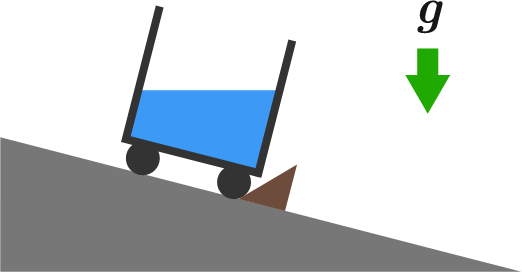A bucket of water on small wheels rests on an inclined plane. While at rest, the calm surface of water experiences the same force at every point, and is perpendicular to the force of gravity.

If the bucket were freely rolling down the plane, what would the water surface look like?

Assume the bucket does not slip, and after some initial sloshing, the water comes to rest.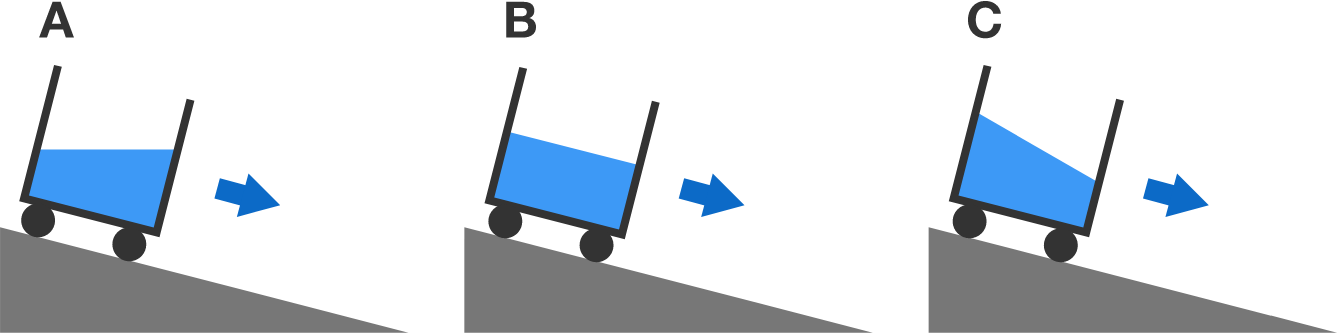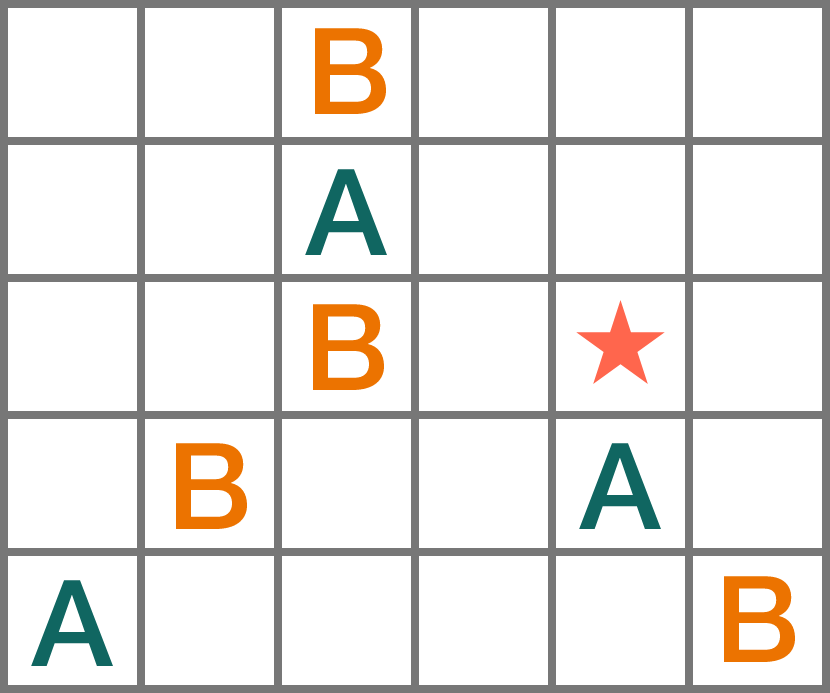Cutting only along the grid lines, we can divide this figure into two congruent parts such that all the As are in one part and all the Bs are in the other.

Will the star end up with the As or Bs?

Note: "Congruent" means "of the same shape and size".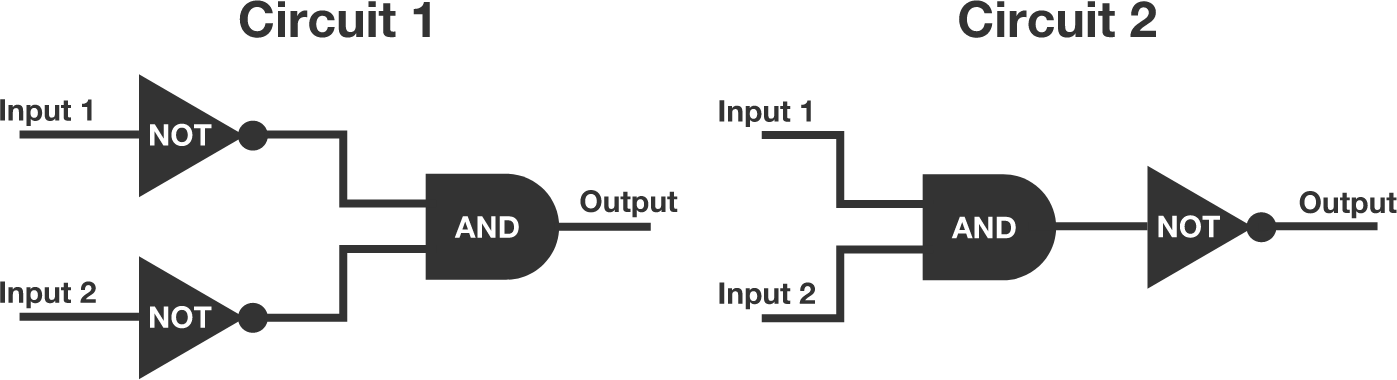Are the two circuit diagrams equivalent?

• An AND gate outputs "ON" only if both inputs are "ON." It outputs "OFF" otherwise.
• A NOT gate outputs "OFF" if the input is "ON," and vice versa.

For integers $M$ and $N$, the following is true:

If $M$ is divisible by $N$, then $M^2$ must be divisible by $N$.

However, the converse of this statement is not necessarily true.

Which of the following statements is false?

×

Problem Loading...

Note Loading...

Set Loading...